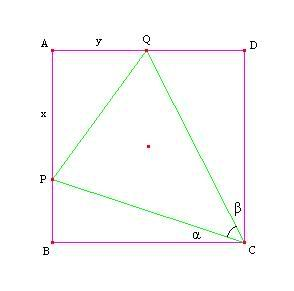## Sunday, April 16, 2006

### Perimeter To Angle? Topic: Geometry/Trigonometry. Level: Olympiad.

Problem: (1986 China TST - #5) Given a square $ABCD$ whose side length is $1$, $P$ and $Q$ are points on the sides $AB$ and $AD$, respectively. If the perimeter of $APQ$ is $2$ find the angle $PCQ$.Solution: Let $AP = x, AQ = y$ and $\angle PCB = \alpha, \angle QCD = \beta$. By the given condition, we have $AP+AQ+QP = x+y+\sqrt{x^2+y^2} = 2$ (1). From this, we find

$x+y = 2-\sqrt{x^2+y^2}$

$(x+y)^2 = 4-4\sqrt{x^2+y^2}+(x^2+y^2)$

$xy = 2-2\sqrt{x^2+y^2}$

Substituting $\sqrt{x^2+y^2} = 2-x-y$ from (1), we have

$xy = 2-2(2-x-y) \Rightarrow 2-x-y = x+y-xy \Rightarrow \frac{2-x-y}{x+y-xy} = 1$ (2).

Note that $\tan{\alpha} = \frac{PB}{BC} = 1-x$ and $\tan{\beta} = \frac{QD}{DC} = 1-y$. Then

$\tan{(\alpha+\beta)} = \frac{\tan{\alpha}+\tan{\beta}}{1-\tan{\alpha} \cdot \tan{\beta}} = \frac{(1-x)+(1-y)}{1-(1-x)(1-y)} = \frac{2-x-y}{x+y-xy}$.

But by (2), we have $\tan{(\alpha+\beta)} = \frac{2-x-y}{x+y-xy} = 1 \Rightarrow \alpha+\beta = 45^{\circ}$.

Hence $\angle PCQ = 90^{\circ}-(\alpha+\beta) = 45^{\circ}$. QED.

--------------------

Comment: Trigonometry can come in handy quite often, especially when dealing with angles. Purely geometric solutions to this problem are a lot more complicated in my opinion; when in doubt, use algebra. The following identity comes in handy on quite a few problems.

--------------------

Practice Problem: Prove that in any triangle we have $\tan{\frac{A}{2}} \cdot \tan{\frac{B}{2}} + \tan{\frac{B}{2}} \cdot \tan{\frac{C}{2}} + \tan{\frac{C}{2}} \cdot \tan{\frac{A}{2}} = 1$.

1.Inscribing a circle into triangle ABC, and using a = x + y, b = y + z, c = z + x, and r, we find that the identity is equivalent to

r^2 / xz + r^2 / xy + r^2 / yz = 1

By Heron's, the area of the triangle A = \sqrt{xyz(x+y+z)} = (x+y+z)r, so A^2 / A^2 = 1 = r^2 (x + y + z) / xyz = 1, which is equivalent to the above identity. QED.

2.Don't you think you should start to slow down a little. USAMO is in two days!!!

3.Tomorrow is my cool down period for the year :).

4.Haha, nice. Well, good luck on the USAMO. You are a great role model. You've worked hard and improved so much in one year.

5.I wonder if this proof is valid:

From the equations AP + AQ + PQ = 2, AP = 1 - PB, and AQ = 1 - QD we get
PQ = PB + QD

This means that triangles PCB and QCD can be "flipped" inward along PC and QC respectively to fill up triangle PCQ.

Then we have 2(alpha + beta) = 90, or alpha + beta = 45 which means alpha + beta = angle PCQ = 45.

6.That's interesting. Intuitively, it makes sense, but I'm not completely sure if I would be able to rigorously prove it. A good idea, though!

7.Hmm, I guess "flipping triangles" inward is not the best way to put it.
8.